# NCERT Solutions For Class 10 Maths Chapter 12 Exercise 12.1- Area related to Circles

Download NCERT Solutions For Class 10 Maths Chapter 12 Exercise 12.1 – Area related to Circles. This Exercise contains 5 questions, for which detailed answers have been provided in this note. In case you are looking at studying the remaining Exercise for Class 10 for Maths NCERT solutions for other Chapters, you can click the link at the end of this Note.

### NCERT Solutions For Class 10 Maths Chapter 12 Exercise 12.1- Area related to Circles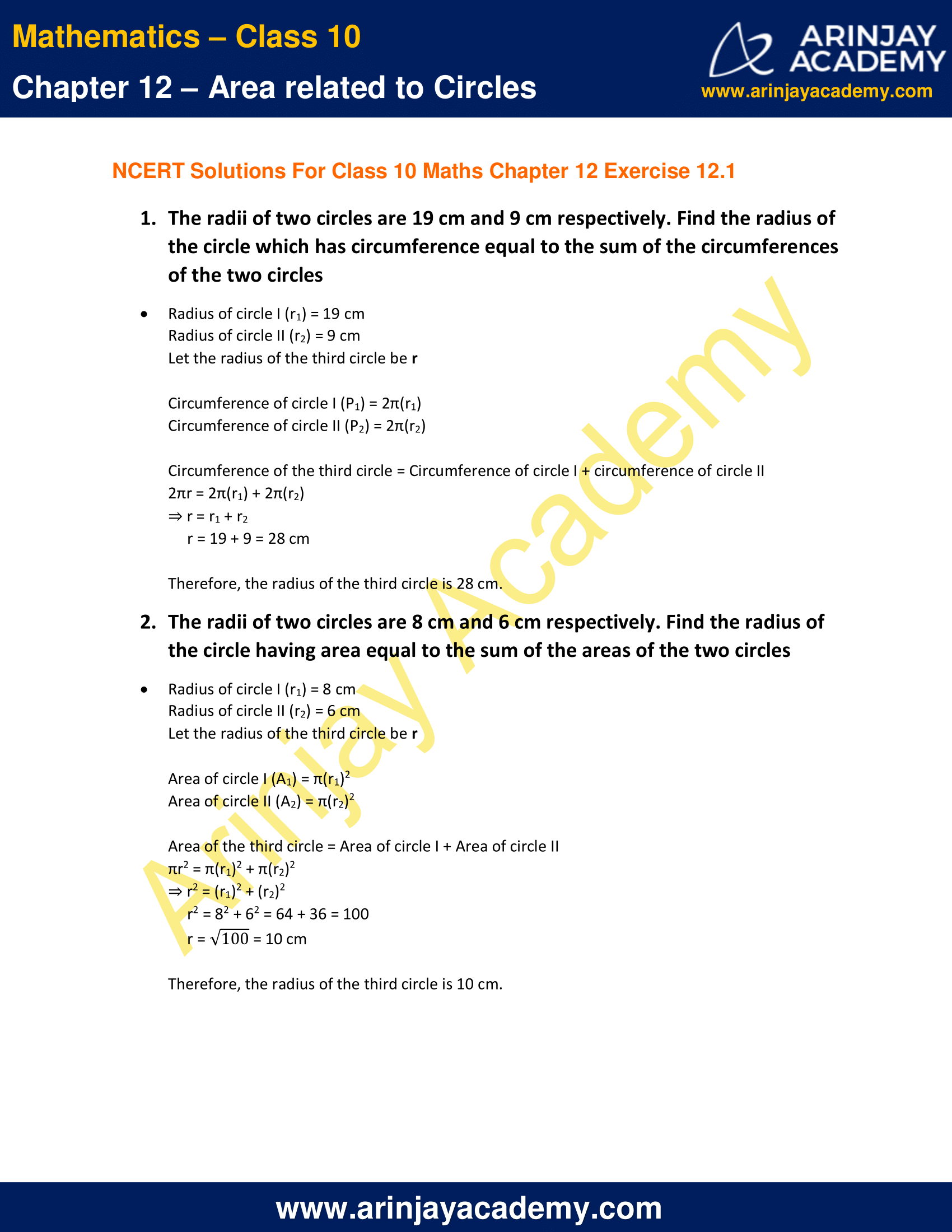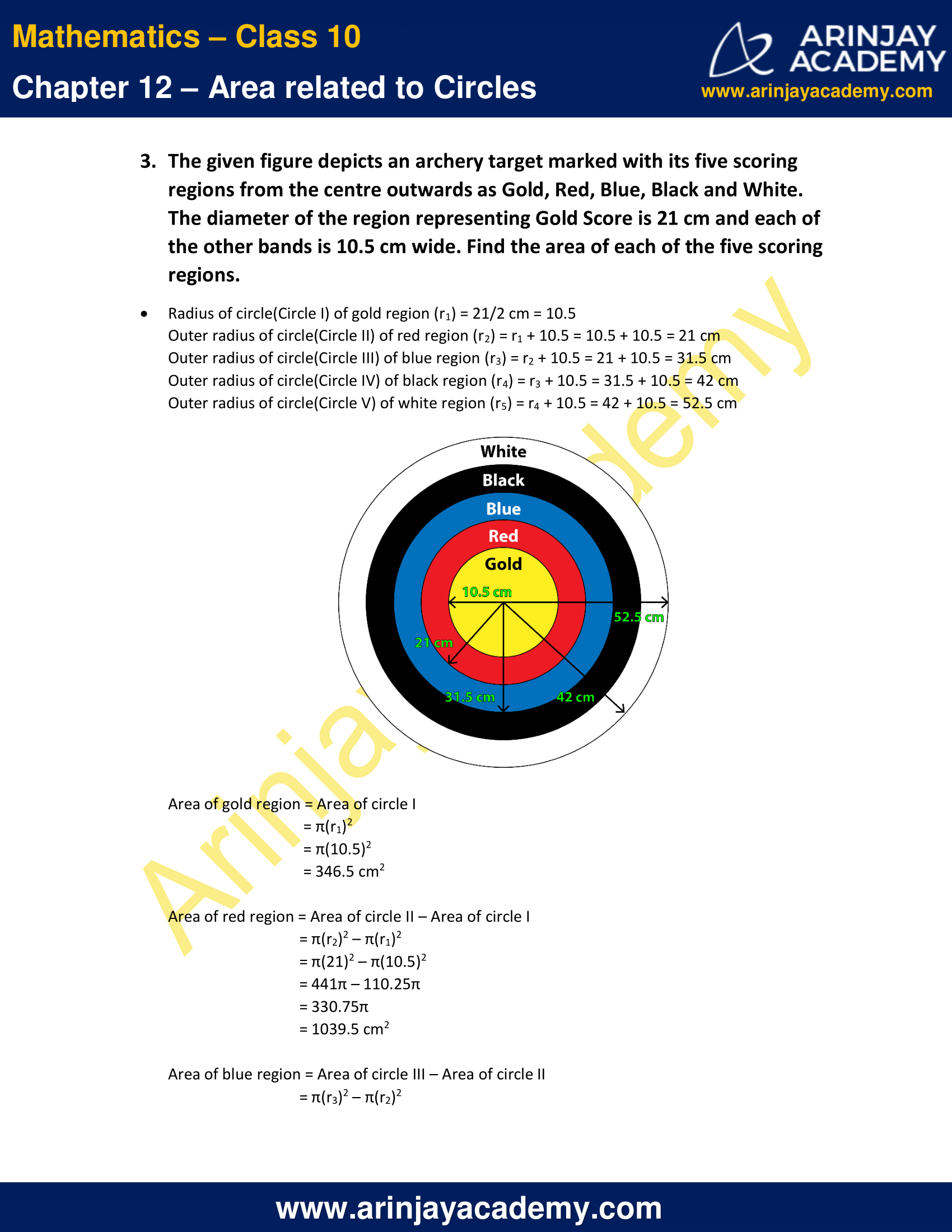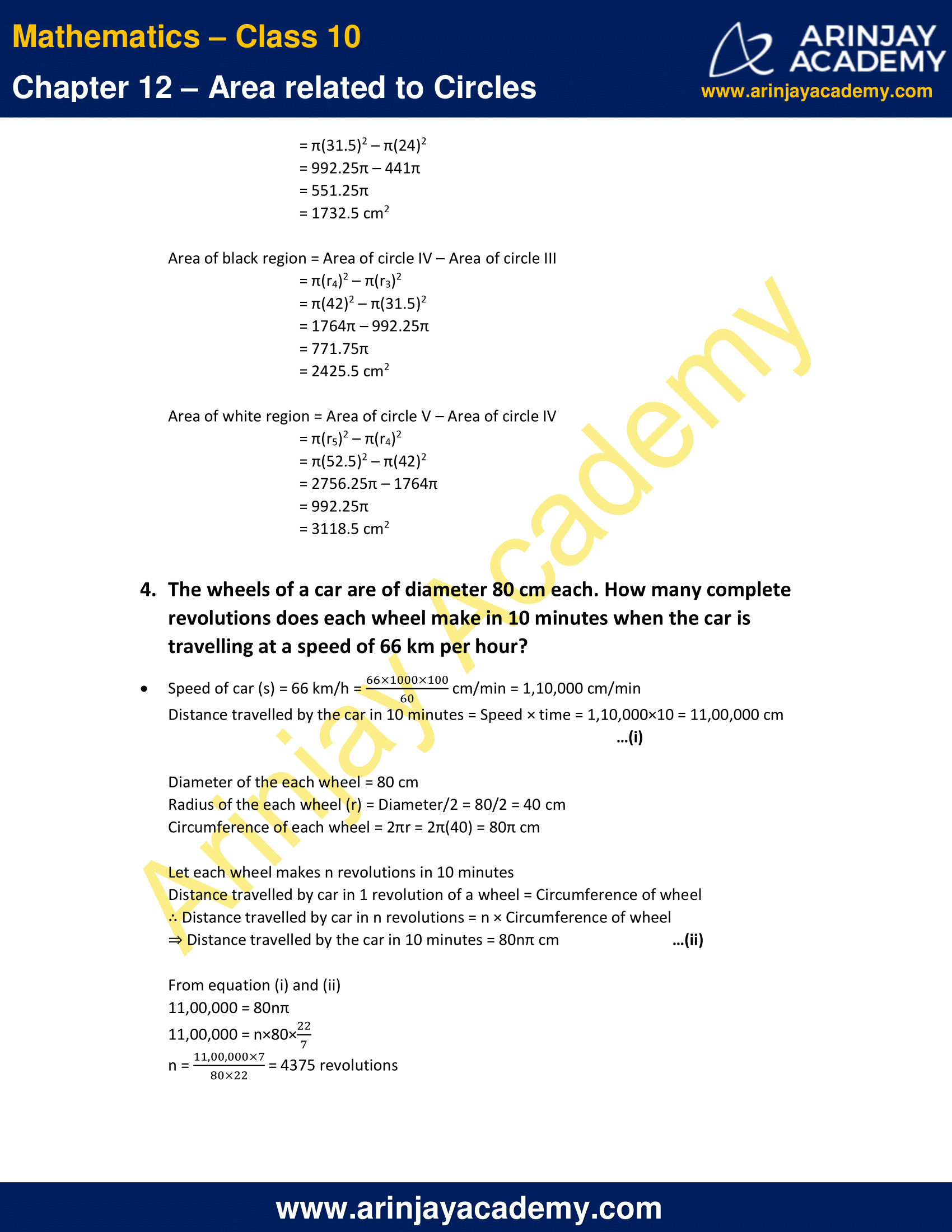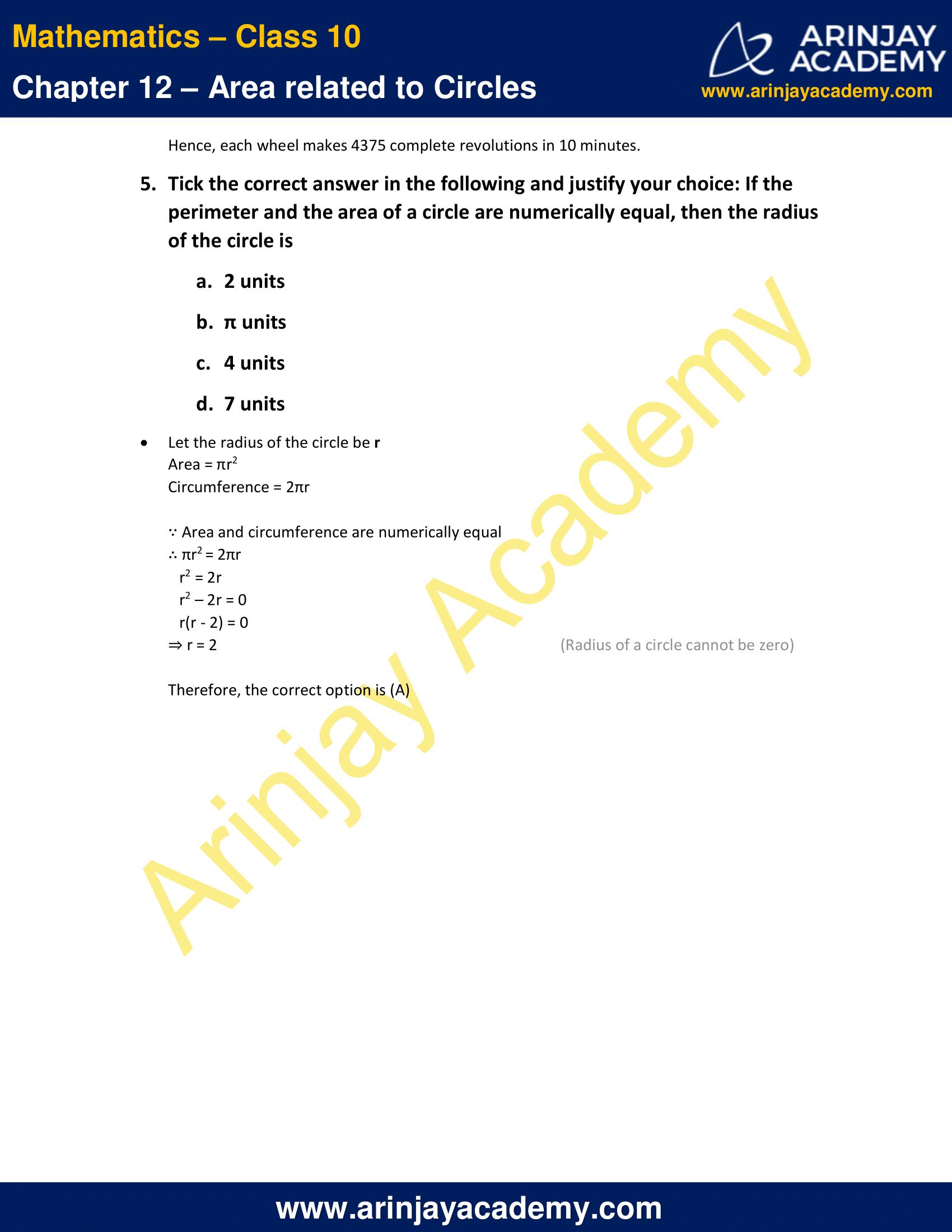NCERT Solutions For Class 10 Maths Chapter 12 Exercise 12.1- Area related to Circles

1. The radii of two circles are 19 cm and 9 cm respectively. Find the radius of the circle which has circumference equal to the sum of the circumferences of the two circles

Solution:

Radius of circle I (r1) = 19 cm
Radius of circle II (r2) = 9 cm
Let the radius of the third circle be r

Circumference of circle I (P1) = 2π(r1)
Circumference of circle II (P2) = 2π(r2)

Circumference of the third circle = Circumference of circle I + circumference of circle II
2πr = 2π(r1) + 2π(r2)
⇒ r = r1 + r2
r = 19 + 9 = 28 cm

Therefore, the radius of the third circle is 28 cm.

2. The radii of two circles are 8 cm and 6 cm respectively. Find the radius of the circle having area equal to the sum of the areas of the two circles

Solution:

Radius of circle I (r1) = 8 cm
Radius of circle II (r2) = 6 cm
Let the radius of the third circle be r

Area of circle I (A1) = π(r1)2
Area of circle II (A2) = π(r2)2

Area of the third circle = Area of circle I + Area of circle II
πr2 = π(r1)2 + π(r2)2
⇒ r2 = (r1)2 + (r2)2
r2 = 82 + 62 = 64 + 36 = 100
r = √100 = 10 cm

Therefore, the radius of the third circle is 10 cm.

3. The given figure depicts an archery target marked with its five scoring regions from the centre outwards as Gold, Red, Blue, Black and White. The diameter of the region representing Gold Score is 21 cm and each of the other bands is 10.5 cm wide. Find the area of each of the five scoring regions.

Solution:

Radius of circle(Circle I) of gold region (r1) = 21/2 cm = 10.5
Outer radius of circle(Circle II) of red region (r2) = r1 + 10.5 = 10.5 + 10.5 = 21 cm
Outer radius of circle(Circle III) of blue region (r3) = r2 + 10.5 = 21 + 10.5 = 31.5 cm
Outer radius of circle(Circle IV) of black region (r4) = r3 + 10.5 = 31.5 + 10.5 = 42 cm
Outer radius of circle(Circle V) of white region (r5) = r4 + 10.5 = 42 + 10.5 = 52.5 cm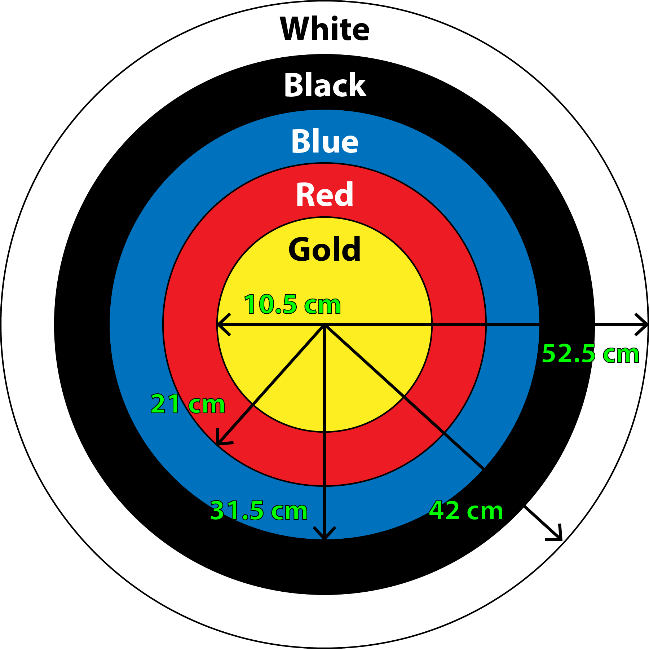Area of gold region = Area of circle I
= π(r1)2
= π(10.5)2
= 346.5 cm2

Area of red region = Area of circle II – Area of circle I
= π(r2)2 – π(r1)2
= π(21)2 – π(10.5)2
= 441π – 110.25π
= 330.75π
= 1039.5 cm2

Area of blue region = Area of circle III – Area of circle II
= π(r3)2 – π(r2)2
= π(31.5)2 – π(24)2
= 992.25π – 441π
= 551.25π
= 1732.5 cm2

Area of black region = Area of circle IV – Area of circle III
= π(r4)2 – π(r3)2
= π(42)2 – π(31.5)2
= 1764π – 992.25π
= 771.75π
= 2425.5 cm2

Area of white region = Area of circle V – Area of circle IV
= π(r5)2 – π(r4)2
= π(52.5)2 – π(42)2
= 2756.25π – 1764π
= 992.25π
= 3118.5 cm2

4. The wheels of a car are of diameter 80 cm each. How many complete revolutions does each wheel make in 10 minutes when the car is travelling at a speed of 66 km per hour?

Solution:

Speed of car (s) = 66 km/h = (66 x 1000 x 100)/60 cm/min = 1,10,000 cm/min
Distance travelled by the car in 10 minutes = Speed × time = 1,10,000×10 = 11,00,000 cm …(i)
Diameter of the each wheel = 80 cm
Radius of the each wheel (r) = Diameter/2 = 80/2 = 40 cm
Circumference of each wheel = 2πr = 2π(40) = 80π cm
Let each wheel makes n revolutions in 10 minutes
Distance travelled by car in 1 revolution of a wheel = Circumference of wheel
∴ Distance travelled by car in n revolutions = n × Circumference of wheel
⇒ Distance travelled by the car in 10 minutes = 80nπ cm  …(ii)
From equation (i) and (ii)
11,00,000 = 80nπ
11,00,000 = n×80×(22/7)
n = (1100000 x 7)/(80 x 22) = 4375 revolutions
Hence, each wheel makes 4375 complete revolutions in 10 minutes.

5. Tick the correct answer in the following and justify your choice: If the perimeter and the area of a circle are numerically equal, then the radius of the circle is

A. 2 units
B. π units
C. 4 units
D. 7 units

Solution:

Let the radius of the circle be r
Area = πr2
Circumference = 2πr
∵ Area and circumference are numerically equal
∴ πr2 = 2πr
r2 = 2r
r2 – 2r = 0
r(r – 2) = 0
⇒ r = 2  (Radius of a circle cannot be zero)
Therefore, the correct option is (A)

NCERT Solutions for Class 10 Maths Chapter 12 Exercise 12.1 – Area related to Circles, has been designed by the NCERT to test the knowledge of the student on the topic – Perimeter and Area of a Circle

Download NCERT Solutions For Class 10 Maths Chapter 12 Exercise 12.1 – Area related to Circles